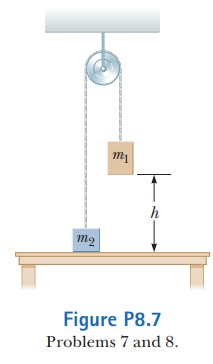# Problem: Two objects are connected by a light string passing over a light, frictionless pulley as shown in Figure P8.7. The object of mass m1 is released from rest at height h above the table. Using the isolated system model, (a) determine the speed of m2 just as m1 hits the table and(b) find the maximum height above the table to which m2 rises.

⚠️Our tutors found the solution shown to be helpful for the problem you're searching for. We don't have the exact solution yet.

###### Problem Details

Two objects are connected by a light string passing over a light, frictionless pulley as shown in Figure P8.7. The object of mass m1 is released from rest at height h above the table. Using the isolated system model,(a) determine the speed of m2 just as m1 hits the table and

(b) find the maximum height above the table to which m2 rises.